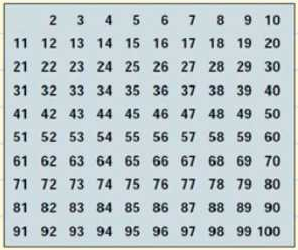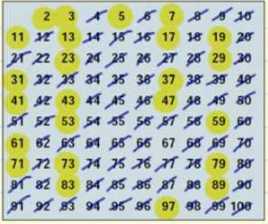Smartick is an online platform for children to master math in only 15 minutes a day

Dec30

# Prime Numbers and Composite Numbers

Today we are going to learn about prime numbers and composite numbers.

#### What is a prime number?

A prime number is a number (greater than 1) that is only divisible by 1 and itself.

#### What are composite numbers?

They are numbers (greater than 1) that are not prime. They are divisible by 1, by itself and by other numbers.

#### Let’s look at an example of a prime and composite number

The number 11 can be written as 1 x 11, but cannot be written as a multiplication problem of any other natural numbers. It only has the factors 1 and 11. Therefore, 11 is a prime number.The number 12 can be written as the 1 x 12, 3 x 4, and 2 x 6. Since 12 is divisible by numbers other than 1 and itself, 12 is a composite number.

#### Prime Numbers Table

We are going to construct the table of prime primes up to 100.• We are going to begin with number 2. Number 2 is a prime number but all multiples of 2 will be composite numbers because they are divisible by 2. We can cross all the multiples of 2 off of our table.
• The next prime number is number 3. Therefore, we can cross all the multiples of 3 off of our table, because they are divisible by 3.
• The next prime number is 5. Therefore, we can cross off all the multiples of 5.
• The next prime number is 7. Therefore, we can cross off all the multiples of 7.
• The next prime number is 11. Therefore, we can cross off all the multiples of 11, which are 22, 33, 44, 55, 66, 77, 88, and 99. All of these had already been crossed off, so we have already finished crossing out all the composite up to 100.

This is our list of prime numbers from 1 to 100. You do not need to learn them by heart, but if you’d like, remember the smaller ones, like 2, 3, 5, 7, 11, and 13:#### Factors of a number

A factor of a number is a value that divides evenly into the number—that is, with no remainder. For example, we are going to calculate the factors of 24. We begin dividing, starting with 1:

• 24 / 1 = 24. Both 1 and 24 are its factors.
• 24 / 2 = 12. 2 and 12 are its factors.
• 24 / 3 = 8. 3 and 8 are its factors.
• 24 / 4 = 6. 4 and 6 are its factors.
• 24 / 5 = 4, with a remainder of 4. Since there is a remainder, 5 is not a factor of 24.
• The next number is 6, but since we already have 6 as a factor of 24, we are finished calculating the factors of 24.

Latest posts by Smartick (see all)

• Olufunke BanjoMay 27 2020, 7:49 AM

Nice

• Janhvi kaharJun 25 2019, 9:31 AM

Nice